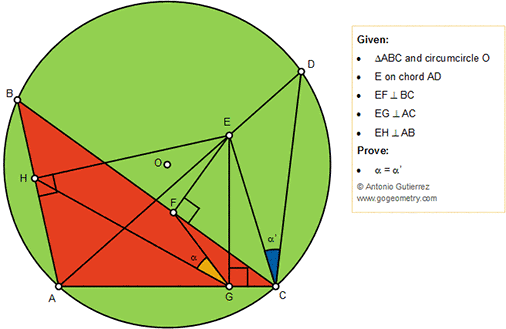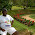## Sunday, November 11, 2018

### Geometry Problem 1399: Triangle, Circumcircle, Perpendiculars, Angles, Congruence, Cyclic Quadrilateral

Geometry Problem. Post your solution in the comment box below.
Level: Mathematics Education, High School, Honors Geometry, College.

Details: Click on the figure below.#### 5 comments:

1.Since CEFG is concyclic,
< ECF = < EGF = x say

Now since AGEC is concyclic,
< EGH = < EAH = < DCB

But < EGH = alpha + x and
< DCB = alpha’ + x and so

alpha = alpha’

Sumith Peiris
Moratuwa
Sri Lanka

2.Solution 2

Since AGEH & ABDC are both concyclic
< HEG = < BDC

So in Tr.s BDC & HEG,
< HEG = < BDC
< EHG = < DBC since each angle = < CAD
Hence < EGH = < DCB

But < EGF = < ECF

So alpha = alpha’

Sumith Peiris
Moratuwa
Sri Lanka

3.Ang BAD = HGF + FGE ( AHEG )
Ang BAD = BCE + ECD ( BD )
Ang FGE = FCE ( FECG )
=> HGF = ECD

4.Unghiul FEG = unghiul FCG (laturi perpendiculare).
De aici FECG circumscriptibil
=> unghiul FGE = FCE (1).
Unghiul AHE = unghiul AGE =90
=> AHEG circumscriptibil
Unghiul HAE = unghiul HGE . (2)
Dar unghiul BAD = unghiul BCD (3)
Din (2) si (3) => unghi BCD= unghi HGE.(4)
Din (1) si (4) => α= α^,
Florin Popa, Comanesti, Romania

5.Is EFGC=cyclic=>α=<DCE=<FGH=α'.